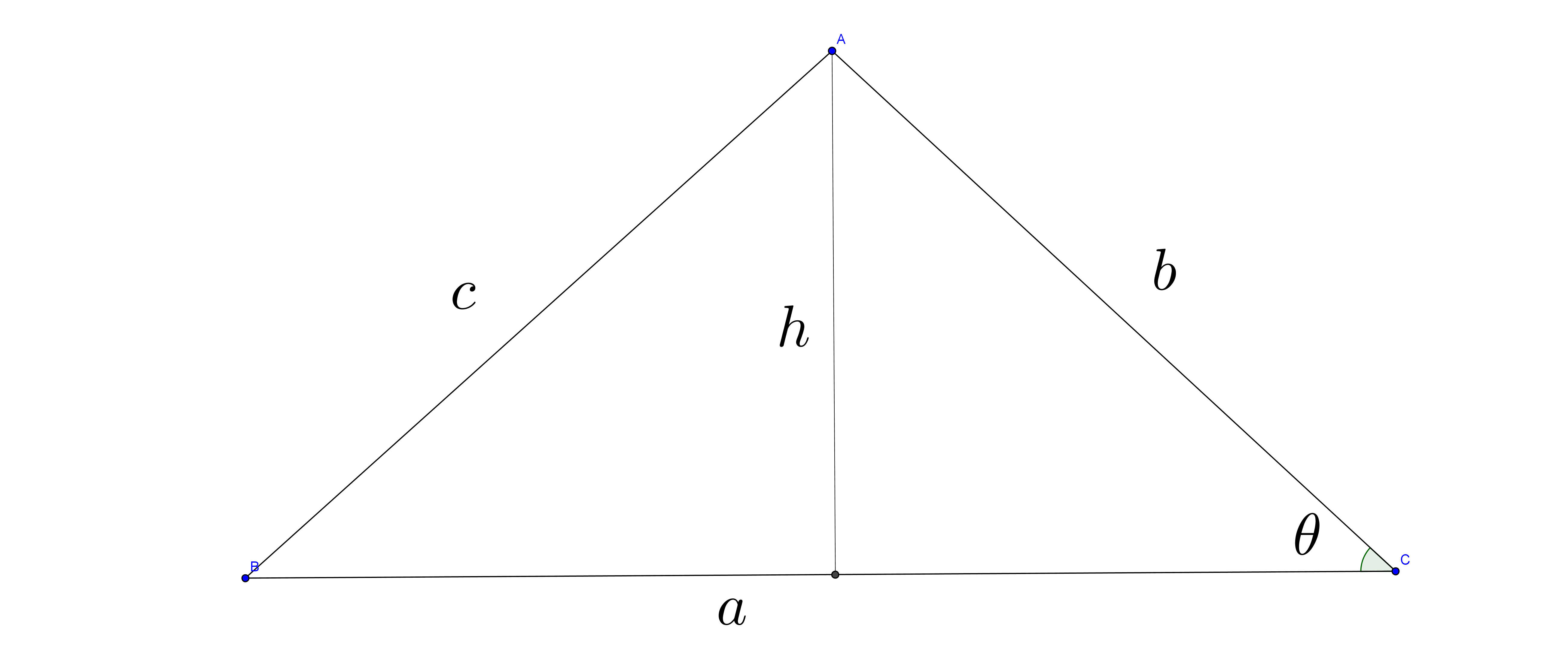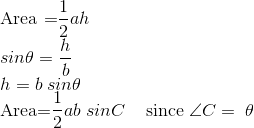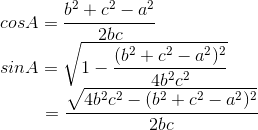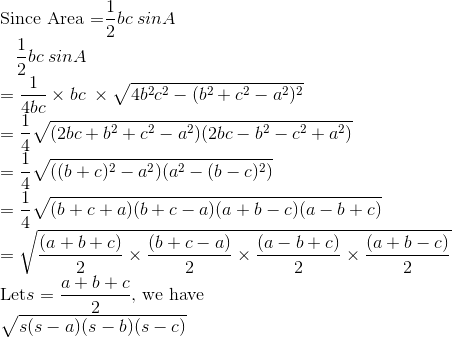Area Formulas

There are two area formulas, one of which uses another to prove. These two formulas can be used in different occasions.

Given two sides and its included angle:If we are given two angles and one side, we can use the law of sine to find the second side, and apply this formula.

If we are given two sides and non-included angle, we can find the third side, then we use the formula below.

Given Three sides only: (Heron's formula)

According to the picture above, we have